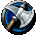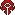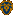## FANDOM

106,204 PagesThis article is a guild progression information page for the Bloodhoof Europe realm (server). The contents herein are entirely player made and in no way represent official World of Warcraft history or occurrences which are accurate for all realms. This page includes a list of guilds that have accomplished a particular task (usually by defeating a major boss). The list should not be taken to be 100% accurate, as it only contains data that has been submitted/added by users of the community. Thus, if you have information you can add, and contribute to the list, it will become more complete.
Legend
x - Not entered
Complete - Endboss down (optional bosses do not count)
Bossname - The guild has downed this and previous bosses of the given instance
Number - The guild has downed this amount of bosses in the given instanceHordedon'tremove Karazhan Gruul's Lair Serpentshrine Cavern The Eye Magtheridon Black Temple Mount Hyjal Tainted Souls: (w) Complete Complete x Complete x x x Blood Cult: (w) Maiden of Virtue x x x x x x Ebrium: (w) Maiden of Virtue x x x x x x The Suffering: (w) Complete Complete x x Complete x x Alpha and Omega: (w) x x x x x x x Avengers: (w) x x x x x x x Bloodstorm: (w) Complete Complete Leotheras the Blind High Astromancer Solarian x x x Censura Divinus: (w) x x x x x x x Cunning Stunts: (w) x x x x x x x Deathless Fire: (w) x x x x x x x DEATHPAX: (w) x x x x x x x De Lage Landen: (w) x x x x x x x Dungeon and Dragons: (w) x x x x x x x Escapism: (w) Complete Complete Complete Complete Complete Complete Complete Even In Death: (w) x x x x x x x Exiled: (w) x x x x x x x Horde Heroes Guild: (w) x x x x x x x Hug Me Harder: (w) x x x x x x x Killer Bunnies: (w) x x x x x x x Nomads: (w) x x x x x x x Northern Darkness: (w) x x x x x x x Pulse: (w) x x x x x x x Pax Imperium: (w) x x x x x x x Sleepwalkers: (w) x x x x x x x StormWatchers: (w) x x x x x x x The Big Pink Outfit: (w) x x x x x x x The Dark Angels: (w) x x x x x x x The Grudge Bearers: (w) x x x x x x x The Immortal Kings: (w) x x x x x x x The Minions of Ensslin: (w) x x x x x x x The Orphanage: (w) x x x x x x x THE WIMP WIPERS: (w) x x x x x x x Toy Soldiers: (w) x x x x x x x Warlords of Bloodhoof: (w) x x x x x x x Zenophobic: (w) x x x x x x xAlliance Karazhan Gruul's Lair Serpentshrine Cavern The Eye Magtheridon Black Temple Mount Hyjal Cause And Effect: (w) Complete Complete Complete Complete Complete Complete Complete Novus Ordo Seclorum: (w) Complete Complete Complete Complete Complete Complete Complete Gatekeepers: (w) Complete Complete Complete Complete Complete 3 2 Pandemonium: (w) Complete Complete Complete Complete Complete 2 2 Evolution: (w) Complete Complete Complete Complete Complete x x Imperial Alliance: (w) Complete Complete Complete Complete Complete Complete Complete Axiom Knights: (w) Complete Complete x x x x x The Hidden Circle: (w) Complete Complete x x x x x Dead Code Elimination: (w) Complete Complete x x x x x Defiance: (w) The Curator / Chess Event x x x x x x Protectorate: (w) Complete Complete x x x x x DCLXVI: Maiden of Virtue x x x x x x Fearless: (w) Shade of Aran x x x x x x Ginnunga: (w) Maiden of Virtue x x x x x x Frostbyte: (w) Complete Complete Hydross Void Reaver x x x Nocturnal Dawn: (w) Moroes x x x x x x Circle of Blades: (w) Attumen the Huntsman x x x x x x Edgeways: (w) Complete Complete The Lurker Below Al'ar x x x Lightwalkers: (w) Attumen the Huntsman x x x x x x Bokkerijders: (w) x x x x x x x Celtic Fist: (w) x x x x x x x Chaotic Eternum: (w) x x x x x x x Chosen Ones: (w) x x x x x x x Clan Bearhawk: (w) x x x x x x x Clan of Chaos: (w) x x x x x x x CrazyDutchGamers: (w) x x x x x x x Cybergings: (w) x x x x x x x Destiny and Elite: (w) x x x x x x x Disconnected: (w) x x x x x x x Drunken Scum: (w) x x x x x x x Equilibrium: (w) x x x x x x x Exile: (w) x x x x x x x Forever Angels: (w) x x x x x x x Horizon: (w) x x x x x x x Imperial Fist: (w) x x x x x x x Judas: (w) x x x x x x x Judean Peoples Front: (w) x x x x x x x Kimen Yahae: (w) x x x x x x x Lords of the Zodiac: (w) Complete Complete Complete Complete Complete Complete Complete Morningstar: (w) x x x x x x x Nightfall: (w) x x x x x x x Order (w) x x x x x x x Reborn: (w) x x x x x x x Rising: (w) x x x x x x x ShadowBlade Rogues: (w) x x x x x x x StormFury: (w) x x x x x x x The Awakening: (w) Complete Complete Complete Complete Complete Complete Azgalor The Chunky Monkeys: (w) x x x x x x x The Fallen Order: (w) x x x x x x x The Omega: (w) x x x x x x x The Outcasts: (w) x x x x x x x UK Thunder: (w) x x x x x x x Utility Crew: (w) x x x x x x x Wild Girls: (w) x x x x x x x Rough NeX: (w) Complete Complete x x x x x Versus: (w) Complete Complete 2 x Complete x x